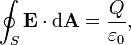Home » Electrostatics » Gauss Theorem – Electrostatics

# Gauss Theorem – Electrostatics

“WHAT IS THE  MAGNITUDE OF ELECTRIC  FIELD IN THE  GAUSSIAN SURFACE OF  A CUBE,AT ITS FACE ,AT ITS CENTRE, AT ITS CORNERS OR AT ANY OTHER POINT INSIDE THE CUBE. ALSO TELL ME THE THE WAY TO KNOW IT.”

Ans:

According to Gauss Theorem, the total electric flux through a closed surface,The Gaussian surface is an imaginary surface. So, for calculating the electric field at a point using Gauss theorem, we have to imagine a Gaussian surface symmetric with the given charge distribution.

I have assumed that there is a point charge Q at the centre of the cube.

At its face (at a point on the face on the line radially outwards from the point charge at the centre of the cube and perpendicular to the face)

The distance is equal to half the length of side of the cube (Say L).

On the corner,

Calculate the distance from the centre of the cube to its corner and replace (L/2) in the above equation with that distance.

This site uses Akismet to reduce spam. Learn how your comment data is processed.

### Hits so far @ AskPhysics

• 2,181,452 hits

### CBSE Exam Count Down

AISSCE / AISSE 2020February 26, 2020
40 days to go.